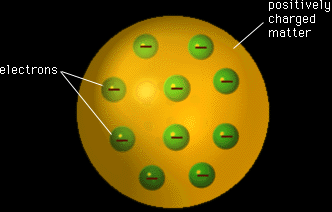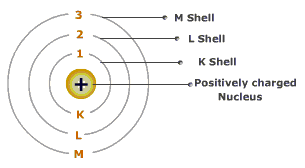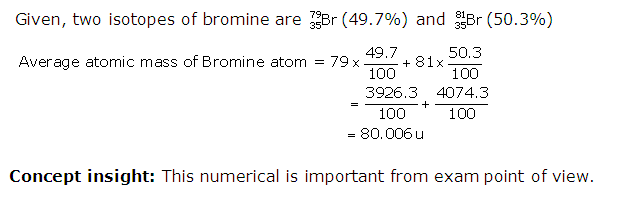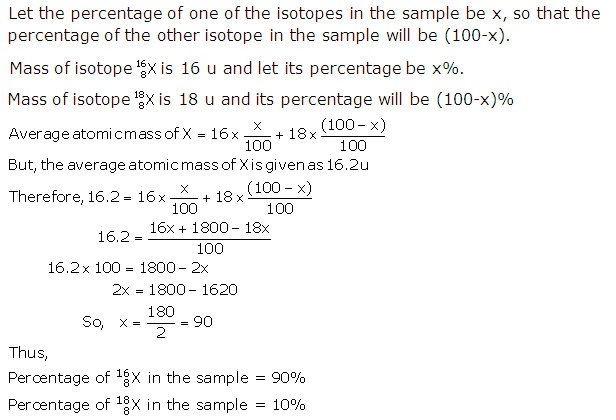Request a call back

# Class 9 NCERT Solutions Chemistry Chapter 4 - Structure Of The Atom

What did Thomson’s proposed model of an atom consist of? What are the three subatomic particles of an atom? Get the correct answers in TopperLearning’s NCERT Solutions for CBSE Class 9 Chemistry Chapter 4 Structure of the Atom. To understand atoms better, you can run through our detailed comparison of Thomson’s model, Rutherford’s model and Bohr’s model.

Go through CBSE Class 9 Chemistry NCERT solutions to learn the definitions of concepts including atomic number, isotopes, isobars and mass number. While these solutions are useful for revision, you may also utilise our concept videos, revision notes and self-assessment resources when preparing to ace your exam.

## Structure Of The Atom Exercise 47

### Solution 1

They are the positively charged radiations which consist of positively charged particles of atoms. Canal rays pass through perforated cathode and then travel towards another cathode in a gas discharge tube. They were given the name Canal rays by E. Goldstein in 1866 who discovered these radiations.

Concept insight: Write the proper definiton of canal rays.

### Solution 2

An atom having one electron and one proton will not carry any charge as the positive charge on the proton will neutralise the negative charge on the electron.

Concept insight: Remember that electron carries a -1 charge and proton carries a +1 charge.

## Structure Of The Atom Exercise 49 - I

### Solution 1

Thomson proposed a model of an atom. According to this model of an atom, an atom consists of a sphere of positive charge. The positive charge in the atom is spread all over like the red edible part of a watermelon, while the electrons are studded in the positively charged sphere, just like the seeds in the watermelon.The negative and positive charges are equal in magnitude. These equal and opposite charges balance each other thus the atom becomes electrically neutral as a whole.

Concept insight: Recall the charges on subatomic particles.

### Solution 2

Proton is the sub-atomic particle present in the nucleus of an atom.

Concept insight: Recall the Rutherfors's model of an atom.

### Solution 3Concept insight: While answering be careful that three shells are asked in the question.

### Solution 4

If a foil of a heavy metal like platinum is used, then the observations in the alpha-particle scattering experiment would be the same as that in the gold foil experiment.

If a foil of a light metal like lithium is used, then the observations in the alpha-particle scattering experiment would not be the same as that in the gold foil experiment.

Concept insight: Recall the proerties of gold.

## Structure Of The Atom Exercise 49 - II

### Solution 1

The three sub-atomic particles of an atom are -
(i) Electrons
(ii) Protons and
(iii) Neutrons

Concept insight: This question is very important from exam point of view.

### Solution 2

Atomic mass of Helium = 4 u
No. of protons = 2

As atomic mass = no. of protons + no. of neutrons
No. of neutrons = At. mass - no. of protons
= 4 - 2 = 2
Concept insight: Recall the correct formula for atomic number and mass number.

## Structure Of The Atom Exercise 50

### Solution 1

 Element At no Electronic configuration K shell M shell L shell Carbon Sodium 6 11 2 2 4 8 1

Concept insight:
Recall that the atomic number of sodium is 11 and that of carbon is 6.

### Solution 2

No. of electrons in K-shell = 2

No. of electrons in L-shell = 8

The total no. of electrons in the atom = 2 + 8 = 10

Concept insight: Recall that K shell has maximum of 2 electrons and L shell has a maximum of 8 electrons.

## Structure Of The Atom Exercise 52 - I

### Solution 1

Chlorine: At. No. of Cl = 17

Its electronic configuration = 2, 8, 7

Valency of Cl = 8 7 = 1

Sulphur: At. no. of S = 16

Its electronic configuration = 2, 8, 6

Valency of S = 8 6 = 2

Magnesium:

At. no. of Mg = 12

Its electronic configuration = 2, 8, 2

Valency of Mg = 2

Concept insight: As we know, when the outermost shell of an atom contains 4 or less than 4 electrons, its valency is equal to the number of valence electrons in the outermost shell and when the outermost shell contains more than 4 electrons, valency of the atom is equal to 8 - no. of valence electrons in the atom.

## Structure Of The Atom Exercise 52 - II

### Solution 1

(i) Atomic number = Number of Protons = 8

(ii) In the given atom, total number of positive charge is equal to the total number of negative charge.

Number of Protons (8) = Number of electrons (8)

So, the charge on the atom will be zero.

Concept insight: Recall the formula of atomic number. Also, remember that the charge on proton is +1 and that on electron is -1.

### Solution 2

Mass number = Number of Protons + Number of neutrons

So, Mass number of oxygen = 8 + 8 = 16

Mass number of sulphur = 16 + 16 = 32

## Structure Of The Atom Exercise 53

### Solution 1

H, D and T are the three isotopes of hydrogen with same atomic number and different mass numbers of 1, 2 and 3 respectively.

 Element Symbols Electrons Protons Neutrons Hydrogen Deuterium Tritium H D T 1 1 1 1 1 1 0 1 2

Concept insight: Recall the mass numbers of each of the isotopes.

### Solution 2

 Isotopes Protons Electrons Neutrons ElectronicsConfiguration Shell K L M N 3517Cl 3717Cl 17 17 17 17 18 20 2 8 7 - 2 8 7 -

 Isobars Protons Electrons Neutrons ElectronicsConfiguration Shell K L M N 4020Ca 4018Ar 20 18 20 28 20 22 2 8 8 2 2 8 8 -

Concept insight: Recall the definitions of isotopes and isobars.

## Structure Of The Atom Exercise 54

### Solution 1

The properties of electrons, protons and neutrons (i.e. nature of their charge, mass and location) are given below in a tabulated form-

 Particle Nature of charge Mass Location Electron Proton Neutron negative (-1) or -1.610-19 C positive (+1) or +1.610-19 C No charge 9.010-31 kg 1.67210-27 kg (1 u) 1.67210-27 kg (1 u) Extra nuclear part Nucleus Nucleus

Concept insight: This question is very important from exam point of view.

### Solution 2

J.J. Thomson attributed the mass of an atom due to electrons and protons which are evenly spread throughout the atom. But this did not agree with observations of Rutherford according to whom the mass is concentrated in a very small space later called nucleus.

Thomson's model of the atom could not explain the results of alpha particle scattering experiment carried out by Rutherford.

Concept insight: Recall the features of J. J. Thomson's model of the atom.

### Solution 3

The major limitation of Rutherford's model of the atom is that it does not explain the stability of the atom. As we know now, when charged bodies move in circular motion, they emit radiations. This means that the electrons revolving round the nucleus (as suggested by Rutherford) would lose energy and come closer and closer to nucleus, and a stage will come when they would finally merge into the nucleus. This makes the atom unstable, which is clearly not the case. The electrons do not fall into the nucleus, atoms are very stable and do not collapse on their own.

Concept insight: Recall the features of Rutherford's model of the atom.

### Solution 4

In order to overcome the objections raised against Rutherford's model of the atom, Neils Bohr put forward his model of the atom. According to Bohr's model of the atom,
1. An atom consists of a small positively charged nucleus at its centre.
2. The whole mass of the atom is concentrated at the nucleus.
3. The volume of nucleus is smaller than the volume of the atom (by a ratio of about 1 : 105).
4. The protons and neutrons of the atom are present in the nucleus.
5. The electrons of the atom, which are negatively charged, revolve around the nucleus in definite circular paths known as orbits or which are designated as K, L, M, N etc. or numbered as (n) = 1, 2, 3, 4 etc. (outward from the nucleus).
6. As each orbit is associated with a fixed amount of energy, these orbits are also known as energy levels.
7. While revolving in diserete orbits, the electrons do not radiate energy. But when an electron jumps from one energy level to another, the energy of the atom changes.### Solution 5

 Feature Thomson's model of an atom Rutherford's model of an atom Bohr's model of an atom 1. Positive Charge (Protons)   2. Negative charge (electrons)     3. Diagrammatic representation       4. Limitation: As per Thomson's model of an atom, an atom consists of a positively charged sphere.   The electrons are embedded in the positively charged sphere of an atom, like the seeds in a watermelon.This model could not explain the results of alpha particle scattering experiment carried out by rutherford. The positive charge is concentrated at the core of the atom, which is called nucleus. The nucleus is surrounded by electrons, and the electrons and the nucleus are held together by electrostatic force of attraction.This model could not explain the stability of the atom. The positive charge is present in the core of the atom, called nucleus. The electrons move in discrete orbits, and each orbit is associ-ated with a definite amount of energy.Advantage: This model explains the stability of atoms.

### Solution 6

The Bohr and Bury scheme for the distribution of electrons in an atom is based on the following rules :
1. The maximum number of electrons which a shell can have is represented by 2n2, where n is the quantum number of that particular energy shell. Thus, the maximum number of electrons in the first four shells are :
1st (K) shell 212 = 2
2nd (L) shell 222 = 8
3rd (M) shell 232 = 18
4th (N) shell 242 = 32
2. The outermost shell, which is also called valence shell, can have a maximum of 8 electrons.
3. The shell next to (or inner to) the outermost shell, which is called the penultimate shell, can accommodate a maximum of 18 electrons, (if permitted by rule 1).
4. Electrons are not accommodated in a given shell unless the inner shells are filled, i.e., the shells are filled in a step-wise manner.

### Solution 7

Valency is defined as the combining capacity of an atom of an element. If an atom has 4 or less than 4 electrons in its valence shell, then valency is equal to the no. of valence electrons. But if it has more than 4 valence electrons, then valency is equal to 8 - no. of valence electrons.

Silicon has atomic number 14 and its electronic configuration is:

 K L M 2 8 4

So, valency of silicon = 8 - 4 = 4

Oxygen has atomic number 8 and its electronic configuration is:

 K L 2 6

So, valency of oxygen = 8 - 6 = 2

Concept insight: Remember that the atomic number of oxygen is 8 and Si is 14.

## Structure Of The Atom Exercise 55

### Solution 8

(i) Atomic number: Atomic number of an atom is the total number of protons present within the nucleus of an atom is known as atomic number.
Example: As sodium atom has 11 protons in its nucleus, its atomic number is 11.

(ii) Mass number: Mass number of an atom is the sum total of the masses of all the nucleons present in the nucleus of an atom, i.e.,
Mass Number = No. of Protons + No. of Neutrons
Example: As a sodium atom has 11 protons and 12 neutrons in its nucleus, its mass number = 11 + 12 = 23.

(iii) Isotopes: Isotopes are the atoms of the same element having same atomic number but different mass number.
Example: Hydrogen has three isotopes 11H, 12H, 13H. The atomic number of all the three is 1, but their mass numbers are 1, 2 and 3 respectively.

(iv) Isobars: Isobars are the atoms of different elements having the same mass number but different atomic numbers.
Example: Mass numbers of calcium and argon atoms are 40, but different atomic numbers 20 and 18 respectively.

Two uses of isotopes are:

(i) An isotope of uranium is used as a fuel in nuclear reactors.

(ii) An isotope of cobalt is used in the treatment of cancer.

Concept insight: Definitions and examples are important from exam point of view.

### Solution 9

Atomic number of Na = 11

No. of electrons in Na atom = 11

So, No. of electrons in Na+ ion = 11 1 = 10

Hence, electronic configuration of Na+ = 2, 8

In Na+, K and L shells are completely filled since K shell can have a maximum of 2 electrons and L shell can have a maximum of 8 electrons.

Concept insight: Remember that when a cation is formed, an electron is removed from the outermost shell of the atom

### Solution 10### Solution 11Concept insight: This numerical is important from exam point of view.

### Solution 12

Atomic number, Z = 3
Distribution of electrons : K = 2, L = 1

So, Valency = 1
The element is Lithium.

Concept insight: This numerical is important from exam point of view.

### Solution 13

As we know, mass number of an atom = No. of protons + No. of Neutrons

So, Mass number of X = 6 + 6 = 12

Mass number of Y = 6 + 8 = 14

As both X and Y have the same atomic number (6) but different numbers (i.e., 12 and 14 repectively), so they are isotopes.

Concept insight: This numerical is important from exam point of view.

(a) F
(b) F
(c) T
(d) T

### Solution 15

(a)(b)(c)(d)### Solution 16

(a)(b)(c)(d)### Solution 17

(a)(b)(c)(d)## Structure Of The Atom Exercise 56

### Solution 18

(d) 2, 8, 1

Concept insight: Recall that the atomic number of sodium is 11.

### Solution 19

First row:

Since atomic no. is 9 so, the element is Fluorine.

Atomic no. = No. of protons = no. of electrons = 9

Mass number = no. of protons + no. of neutrons = 9 + 10 = 19

Second row:

Since atomic no. is 16 so, no. of protons = no. of electrons = 16

No. of neutrons = Mass no. - no. of protons = 32 - 16 = 16

Third row:

No. of protons = Atomic no. = 12

So, the element is Magnesium.

No. of electrons = no. of protons = 12

No. of neutrons = Mass no. - no. of protons = 24 - 12 = 12

Fourth row:

No. of protons = Atomic no. = 1

So, the element is Deuterium.

No. of electrons = no. of protons = 1

No. of neutrons = Mass no. - no. of protons = 2 - 1 = 1

Fifth row:

No. of protons = Atomic no. = 1

The element is Protium since the mass number is 1.

 Atomic number Mass number Number of neutrons Number of protons Number of electrons Name of the atomic species 9 16 12 1 1 19 32 24 2 1 10 16 12 1 0 9 16 12 1 1 9 16 12 1 0 Fluorine Sulphur Magnesium Deuterium Protium

Concept insight: For asnwering this question, recall the definitions of atomic number and mass number.